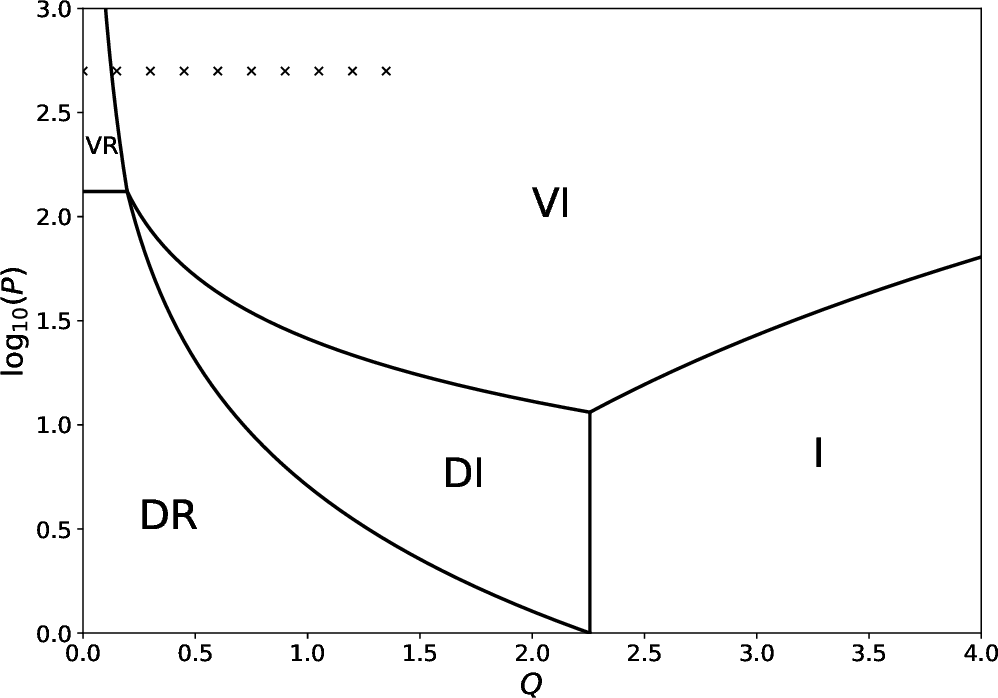# Response Regimes in Tokamak Fusion Reactors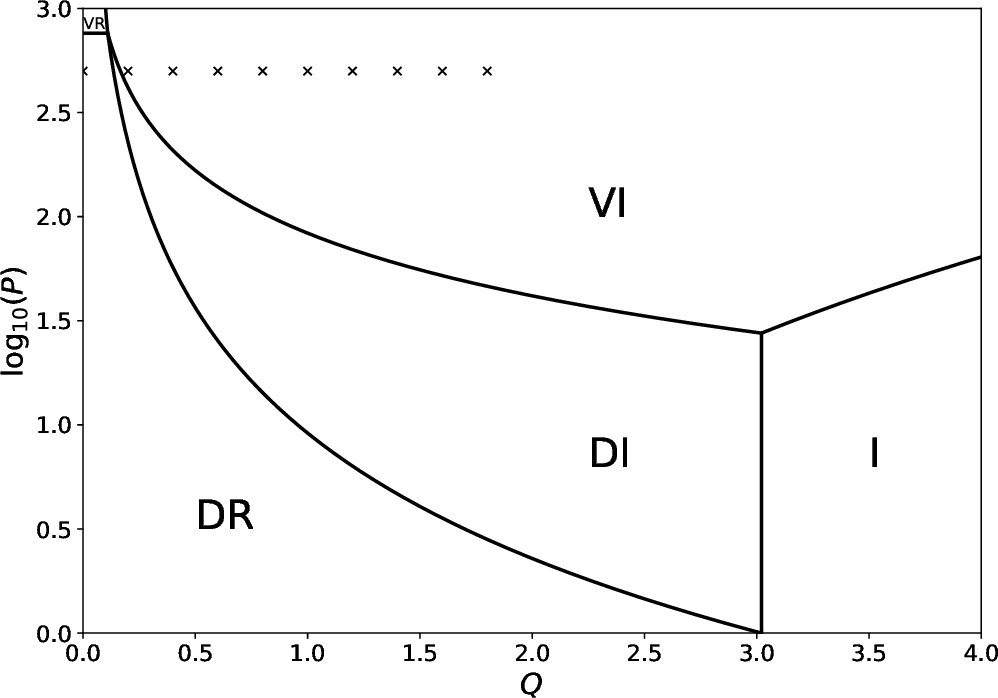Figure 5.3 shows the linear response regimes in a low-field tokamak fusion reactor. The boundaries between the regimes are calculated with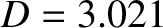. (See Table 5.1.) If we assume that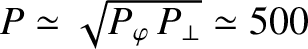and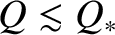, which implies that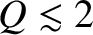(see Table 5.1), then it is clear from the figure that the relevant response regimes are the viscous-inertial and the diffusive-resistive. Note that the respective inequalities,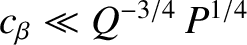and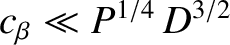, that must be satisfied in order to justify our neglect of the ion parallel dynamics in the layer equation are very easily satisfied in the viscous-resistive and resistive-diffusive regimes. (See Section 5.7 and Tables 5.1 and 5.2.)

Figure 5.4 shows the linear response regimes in a high-field tokamak fusion reactor. The boundaries between the regimes are calculated with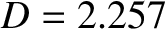. (See Table 5.1.) If we assume thatand, which implies that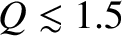(see Table 5.1), then it is clear from the figure that the relevant response regimes are the viscous-inertial and the viscous-resistive. Note that the respective inequalities,and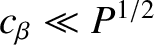, that must be satisfied in order to justify our neglect of the ion parallel dynamics in the layer equation are very easily satisfied in the viscous-inertial and viscous-resistive regimes.